# 高频共模电流、电压和阻抗的测量（中）## 采用磁环解决方案测量CM电流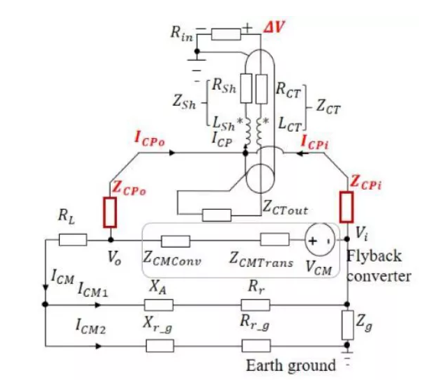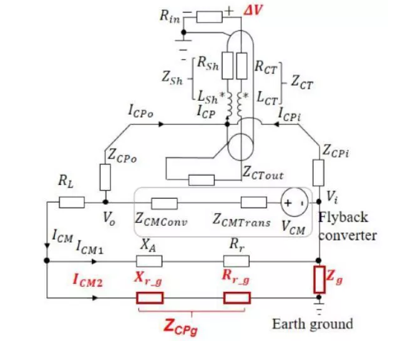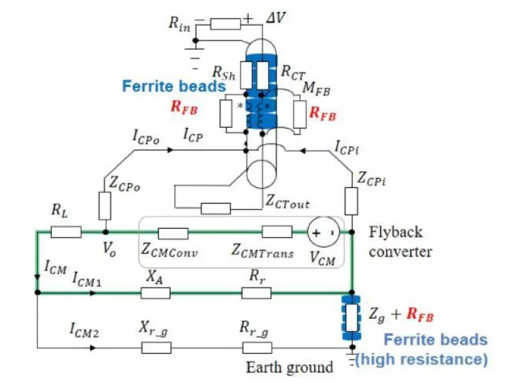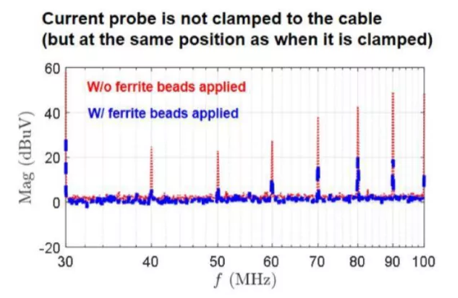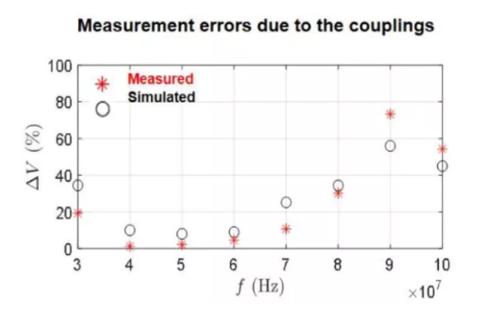## 测量反激式变换器中的共模阻抗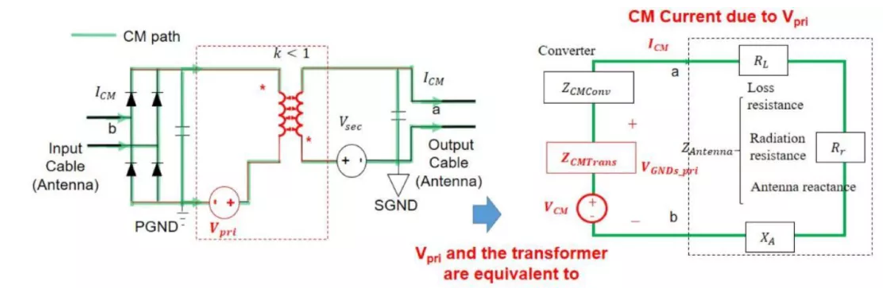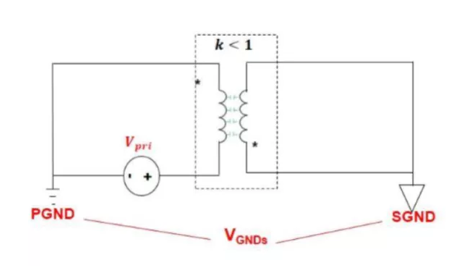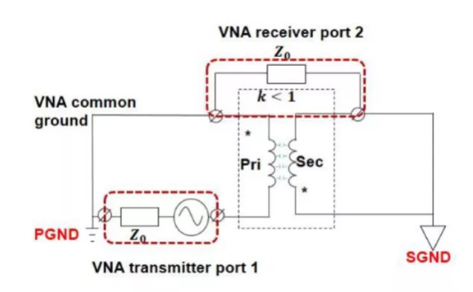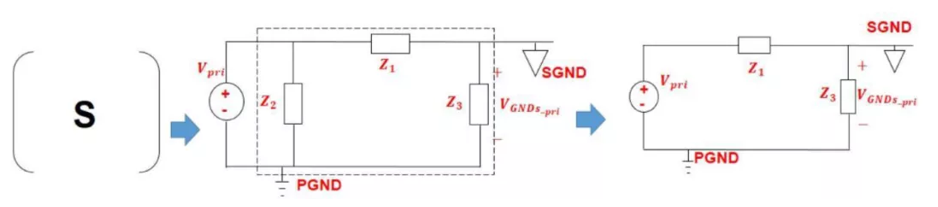$$V_{CMPri} = V_{Pri} \times CMTG_{Pri} = V_{Pri} \times\frac{Z_3}{Z_1+Z_3}$$

$$Z_{CMTransPri} = \frac{Z_3}{Z_1+Z_3}$$